Reach Us+44-1522-440391
Count Data Analysis in Randomised Clinical Trials | OMICS International
Journal of Biometrics & Biostatistics

Like us on:

All submissions of the EM system will be redirected to Online Manuscript Submission System. Authors are requested to submit articles directly to Online Manuscript Submission System of respective journal.

Count Data Analysis in Randomised Clinical Trials

Jakobsen JC1,2*, Tamborrino M3, Winkel P1, Haase N4, Perner A4, Wetterslev J1 and Gluud C1

1Copenhagen Trial Unit, Centre for Clinical Intervention Research, Department 7812, Rigshospitalet, Copenhagen University Hospital, Copenhagen, Denmark

2Emergency Department, Holbaek Hospital, Holbaek, Denmark

3Institute for Stochastics, Johannes Kepler University Linz, Austria

4Department of Intensive Care, Rigshospitalet, University of Copenhagen, Denmark

*Corresponding Author:
Jakobsen JC
Department 7812, Rigshospitalet, Copenhagen University Hospital
Blegdamsvej 9, DK-2100 Copenhagen, Denmark
Tel: +45 2618 6242
E-mail: [email protected]

Received date: April 24, 2015; Accepted date: May 29, 2015; Published date: June 05, 2015

Citation: Jakobsen JC, Tamborrino M, Winkel P, Haase N, Perner A, et al. (2015) Count Data Analysis in Randomised Clinical Trials. J Biomet Biostat 6: 227. doi: 10.4172/2155-6180.1000227

Copyright: © 2015 Jakobsen JC, et al. This is an open-access article distributed under the terms of the Creative Commons Attribution License, which permits unrestricted use, distribution, and reproduction in any medium, provided the original author and source are are credited.

Visit for more related articles at Journal of Biometrics & Biostatistics

Abstract

Choosing the best model for analysis of count data in randomised clinical trials is complicated. In this paper, we review count data analysis with different parametric and non-parametric methods used in randomised clinical trials, and we define procedures for choosing between the two methods and their subtypes. We focus on analysis of simple count data and do not consider methods for analyzing longitudinal count data or Bayesian statistical analysis. We recommend that: (1) a detailed statistical analysis plan is published prior to access to trial data; (2) if there is lack of evidence for a parametric model, both non-parametric tests (either the van Elteren test or the Tadap2 test, based on an aligned rank test with equal stratum weights) and bootstrapping should be used as default methods; and (3) if more than two intervention groups are compared, then the Kruskal–Wallis test may be considered. If our recommendations are followed, the risk of biased results ensuing from analysis of count data in randomised clinical trials is expected to decrease.

Keywords

Count data; Randomised clinical trials; Statistical methodology

Background

It is often optimal to use a dichotomous patient relevant outcome (for example, all-cause mortality) as primary outcome in a randomised clinical trial. Count data outcomes may be used as primary outcomes and are often used as secondary outcomes. Two types of count data are generally used in randomised clinical trials to investigate intervention effects: (a) observations expressed as discrete positive values arising from counting rather than ranking (for example, numbers of serious adverse events or days of intensive care) ; (b) number of events occurring in time intervals or space, with models focusing on the rate at which the events occur (for example, number of adverse events per day) .

It is possible in some circumstances (often with large number of counts) to analyse count data as continuous data (for example, using ANCOVA). However, it is our experience that count data in randomised clinical trials may rarely be validly analyzed as continuous data because the underlying statistical assumptions are rarely met. Several specific methods are available for analyzing count data, but we may fail in choosing the best method. For example, the underlying assumptions for choosing the model might not be fulfilled and the model may not fit the data properly. Furthermore, comparing groups with multiple tests showing different results will increase the risk of having at least one false positive significant result due to ‘play of chance’ (type I error) and makes it possible for trialists to choose specific tests based on whether the test show significance or not [3,4]. Therefore, a detailed procedure on how to choose the most reliable method for analysis of count data should be developed and published prior to access to the trial data .

In the following we present a review of different parametric and non-parametric methods to analyse count data in randomised clinical trials. We highlight their strengths and limitations, and define a procedure for choosing between the two methods with subtypes, depending on the data for analysis. The aim of our review is to provide guidance trialists in analysis of count data outcomes. We do not present or discuss methods for analysis of longitudinal count data or methods for Bayesian statistical analysis which are separate topics [1,6,7].

Methods

Based on a comprehensive literature search in PubMed and methodological and statistical considerations, selected experienced trialists, methodologists, and statisticians considered a variety of different models for analysing count data in randomised clinical trials.

Results

Design of the count data outcome measure

Our study of the literature, made us infer that the first thing to do is to consider how to design the count data outcome. For example, ‘Number of exacerbations’ may be analysed as count data without changing the design of the outcome. However, ‘days of hospital admission’ can, for example, be a problematic outcome in case of early mortality among the patients. In such a case, the patients who die early would contribute with low values for the number of days of admission, which otherwise would be perceived as a beneficial result. Furthermore, it can be difficult to gather count data observations within long-term periods of time, so for practical reasons data may only be collected within a limited time period. It is, therefore, often advantageous to design count data outcomes as, for example, ‘days alive and out of hospital within 90 days’, or to use rates instead of counts. If the observation period differs among the participants, for example when the first randomised participants are observed for a longer period of time than the participants randomised later during the trial, then the data from each participant can be adjusted according to the length of the observation period for each participant. ‘Days alive and out of hospital’ may be presented as the percentage of days alive spent outside the hospital within a specified observation period considering not only the index admission, i.e., the outcome is analysed as a rate.

Simple Parametric Tests

Unadjusted count data may be analysed by two sample inferential procedures (for example, a Student’s t-test).

The generalised parametric linear model

In many trials we want to adjust the analysis of an outcome using covariates in a regression analysis. It may be essential to adjust for stratification variables and potentially other design variables [8-10]. Therefore, it is preferable, if possible, to analyse count data using a parametric regression model.

The generalized linear model is a generalization of the linear regression model allowing response variables not to be normally distributed . Moreover, the generalized linear model allows the linear model to be related to the response variable via a link function and the magnitude of the variance of each measurement to be a function of its predicted value . Hence, a generalized linear model is made up of a linear predictor: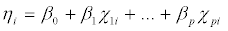And two functions:

A link function (g(μi)) that describes how the mean, E (Yi)=μi, depends on the linear predictor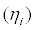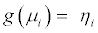• A variance function (var(Yi)) that describes how the variance, var(Yi), depends on the mean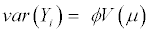A common way to analyse count data is to use Poisson regression models which are generalised linear models based on the Poisson distribution function with the logarithm as the link function . A Poisson distribution is characterised by equal mean and variance and is right-skewed, i.e., the right tail of the distribution is longer . An underlying assumption is that occurrences (count data events) are assumed to be independent . As the mean increases, the skewness decreases and the distribution becomes more symmetric . Depending on the properties of the observed data, for example, the number of observed zeros and the relation between the empirical mean and variance, other underlying distributions may be assumed: (a) a negative binominal may be used when the variance exceeds the mean and the observations are not independent ; (b) zero inflated (Poisson and negative binominal) models may be used when the count outcome exhibits more variation than that which is accommodated by the postulated model due to a preponderance of zero counts ; and (c) zero truncated (Poisson and negative binominal) models may be used when the value zero cannot occur .

The strengths of using these generalised linear models are that trial results may be adjusted for multiple stratification variables, and multiple intervention groups may be compared. Furthermore, odds ratios, incidence rate ratios, ratios of rate ratios, all with confidence intervals may present evidence of the intervention effects with a proper indication of uncertainty [8,9].

Standard approaches for both model checking and model selection need to be applied when choosing the model with the best data fit . Analysis of residuals, for example scatterplots of residuals versus predicted values, is commonly used for model checking in regression analyses. If two or more models provide a good fit of the data via model checking, then the Akaike information criterion (AIC) and the Bayesian information criterion (BIC) can be applied for model selection, provided that the likelihood function (L) of the model is available . In particular, we have AIC=-2 log L+2p and BIC=-2 log L+plogT, where log L denotes the fitted log-likelihood of the model, p is the number of parameters, and T is the number of observations. Then, among the competing models, we shall choose the one with the smallest BIC and AIC (with most importance to BIC).

In practice, to deal with these models can be difficult. If the count data outcomes are designed as we recommend in this review (see ‘Design of the count data outcome’) , then model checking will often show that the underlying assumptions of these generalised linear models are not fulfilled (Table 1) . For example, a common problem arising with Poisson regression models is over dispersion, i.e., the count data outcomes exhibit more variation than the variation expected from the underlying model .

Model type Deviance/d.f. Pearson Chi-square Standardised deviance residuals
/d.f. Mean difference and p-value for H0: mean difference=0 Median p-value for H0: normal distribution (via Kolmogorow-Smirnow test) p-value of intervention
Poisson 1.88 1.28 -0.141, 0.283 <0.0005 0.92
p=0.004
(link function: log) -1.47 -1.06 (-0.083; (-0.000) (<0.0005) -0.56
p<0.0005)
Negative binomial (link function: log) 0.11 0.045 -0.035; 0.068 <0.0005 0.79
p=0.004
-0.097 -0.047 (-0.093; (-0.027) (<0.0005) -0.7
p<0.0005)

Table 1: Data from the Scandinavian starch for severe sepsis/septic shock (6S) trial on the rate of dialysis-free days within 90 days following randomisation.

Generalised linear mixed models (GLMMs), for example, the Poisson generalised linear mixed model, extend the class of generalised linear models and they may be useful in dealing with over dispersion [2,14]. Generalised linear mixed models combine the properties of two statistical frameworks, i.e., linear mixed models (which incorporate random effects) and generalised linear models (which handle nonnormal data by using link functions and exponential family (a set of probability distributions having a certain form) (for example, Poisson or negative binomial distributions) . The use of the generalised linear mixed models allow for some degree of non-independence among observations, for example, when analysing longitudinal or clustered data [2,14,15]. The price to pay for these advantages is a low mathematical tractability since the integration over the random effect is not known in an analytic form, and mathematically-advanced algorithms such as the Markov chain Monte Carlo methods have to be used. Moreover, checking the underlying assumptions is often not possible [2,14,16].

Sandwich covariance matrix estimators are a popular tool in applied regression modelling . Employing estimators for the covariance matrix based on this sandwich form can make inference for the parameters more robust against certain model misspecifications. However, sandwich covariance matrix estimators should not be used for every model in every analysis . First, if the model is correctly specified, the use of sandwich estimators leads to loss of power . Second, if the model is not correctly specified, the sandwich estimators are only useful if the estimates of the parameters are still consistent, i.e., if the misspecification does not result in bias .

When analysing longitudinal data or data of a cluster randomised trial, another extension to the generalised linear model, namely generalised estimating equations (GEE) might be used . GEE avoids modelling the within-patient (or cluster) covariance structure by treating the covariance structure as a nuisance . Hence, the covariance structure does not need to be specified correctly in order to get valid results .

Non-parametric methods

When data are not normally distributed, non-parametric analytic methods ought to be considered. A number of non-parametric methods may be used to analyse count data. The Wilcoxon rank sum test, also known as the Wilcoxon-Mann-Whitney test or the Mann-Whitney U test, is a commonly used non-parametric test to detect differences in the distributions of the response of interests between two treatments . In general, when data are normally distributed, non-parametric tests have lower power than parametric tests, but the power loss with, for example, the Wilcoxon rank sum test compared to that of the t-test is often limited . When normality is violated, the Wilcoxon rank sum test can be three or four times more powerful than the independent samples t-test .

It is generally recommended to adjust for stratification variables (for example, for the effect of clinical site in a multi-center trial) in the primary analysis of a randomised clinical trial [8,9,18]. As it is not possible to adjust for stratum effects via the Wilcoxon rank sum test [18,20], we propose to use alternative tests. One option is the van Elteren test, which is a stratified Wilcoxon rank sum test [18,20].

The van Elteren test essentially tests :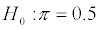Where the competing probability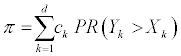and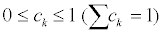are weights and Xk and Yk are random variables with distribution Fk and Gk.

Simulations have shown that the van Elteren test is more efficient by having higher power and lower risk of type I error than the Wilcoxon rank sum test when the number of strata is relatively low (below 50) . The Wilcoxon rank sum test seems to be more efficient than the van Elteren test when the stratum effects are small and at the same time a large number of strata are used . However, unless the sample size is very large, the number of strata in a randomised clinical trial should be much less than 50 . The van Elteren test is available in different commercial statistical programs, for example STATA  and SAS .

A recent simulation study has shown that other non-parametric tests may outperform the van Elteren test in terms of statistical power, especially if the effects across strata differ significantly . Among these tests, the best one in terms of power is the so-called Tadap2 which uses the aligned rank test with equal stratum weights in a sequential manner via an adaptive multiple testing strategy . However, presently the Tadap2 test is not included in any standard statistical software, except for a code implemented in the R-software , kindly provided by Mehrotra et al. . Further comparative studies examining the strengths and limitations of these different non-parametric tests are needed, but both the van Elteren and the Tadap2 tests seem equally reliable methods for analysing count data.

Using the van Elteren and the Tadap2 tests, it is possible to nonparametrically assess the hypothesis that the stratified effects are statistically the same. It may also be relevant and informative to estimate the difference between the medians (and/or the means) of the two interventions. To obtain a non-parametric estimate of the confidence interval of the intervention effect, different techniques may be applied [25,26]. Among others, we propose bootstrapping which estimates the confidence interval by sampling randomly from the observed data [20,25]. A limitation of using bootstrapping to obtain a non-parametric estimate of the confidence interval of the intervention effect is that the uncertainty of the estimated non-parametric confidence interval might be considerable if the sample size is limited or small. Other valid methods which can provide confidence intervals are the Kendall’s τa, Somers’ D, and the Hodges–Lehmann median difference methods .

There are two limitations in using the van Elteren and the Tadap2 tests. First, it is only possible to adjust the data for one stratification variable, for example clinical site in a multicenter trial. Second, only two intervention groups can be compared using these tests. If more than two intervention groups need to be compared, then the Kruskal- Wallis test, which is the non-parametric version of the one-way analysis of variance test (ANOVA), may be used. A stratified version of the Kruskal-Wallis test is available in R .

Discussion

In contrast to most observational studies , obtaining a perfect model fit when analysing count data outcomes of randomised clinical trials is often not necessary. A given trial population might not be similar to the population of another trial studying the same disease, intervention, and outcomes. If data driven transformations are used to optimise a model fit in a given trial, the same transformations may not lead to an optimal model fit in future trials assessing the effects of the same intervention on comparable populations. It will, therefore, be difficult to replicate a given trial result if outcomes or covariates are transformed (e.g., square root, square, or inverse) in different statistical ways to optimise a model fit. Furthermore, the primary aim in a randomised clinical trial is to assess whether an intervention works or not, and precise estimations of covariates, coefficients, etc., might not be of primary interest. On the other hand, to ensure the validity of the trial results and under some circumstances to optimise their power, choosing correctly the optimal statistical method is essential. The optimal choice of tests of assumptions and analysis methods should bring about optimal balance between obtaining a model fit and using a methodology which would allow the trial results to be replicated and generalised. We believe that our present review may help trialists to find this balance.

The aim of our review was to provide a simplified, valid, hands-on guide for trialists faced with simple count data. We have deliberately not included in-depth descriptions of theoretical issues and statistical details, and we have not included considerations of longitudinal count data analysis or Bayesian statistical analysis [1,6,7]. Therefore, we have focused on the most common aspects of count data analysis in randomised clinical trials. An overview of the strengths and limitations of using simple count data in analysis methods is presented in Table 2.

Analysis method Strengths Limitations Notes
The van Elteren test and the Tadap2 tests No underlying assumptions about the distribution of the observed data are necessary.
It is possible to adjust for stratum effects.
Only possible to adjust the data for one stratification variable. Only two intervention groups can be compared. The Tadap2 test is available only in a code written in R. Non-parametric test. Often the optimal choice of analysis method.
Bootstrapping No underlying assumptions about the distribution of the observed data are necessary. The uncertainty of the non-parametric confidence interval provided via bootstrapping could be large if it is based on a limited number of observations. Non-parametric method to estimate the confidence interval of, e.g., means difference or median difference of two treatments.
The Kruskal–Wallis tests Multiple intervention groups can be compared.
No underlying assumptions about the distribution of the observed data are necessary.
The stratified version of the test is available in R only. Non-parametric method.
A valid method but only if more than two groups have to be compared.
The Wilcoxon rank sum test No underlying assumptions about the distribution of the observed data are necessar Not possible to adjust for stratum effects. Non-parametric test. The van Elteren test is often a better choice.
Generalised linear model Trial results can be adjusted for multiple stratification variables.
Odds ratios and confidence intervals can demonstrate the intervention effects.
Multiple intervention groups can be compared.
It can be impossible to deal with some data due to overdispersion. Model checking will often show that the underlying assumptions of the models are not fulfilled. Parametric method. Often not an optimal choice of analysis method.
Generalised linear mixed model Trial results can be adjusted for multiple stratification variables.
Odds ratios and confidence intervals can demonstrate the intervention effects.
Multiple groups can be compared.
Can often handle overdispersion.
Complicated analysis. Checking the underlying assumptions is not easy. Parametric method. Often not an optimal choice of analysis method.

Table 2: An overview of the strengths and limitations when using the different count data analysis methods.

As with all statistical analysis, analysis of count data ought to rest on transparently published, detailed statistical plans for the conduct of the analyses . Such detailed analysis plans ought to be formulated before the data are collected or at least before investigators or statisticians get access to the trial data . If such detailed statistical analysis plans demonstrate defects during the conduct of the analysis, then, of course, the plans must be transparently amended and reported .

Conclusion

The design of the count data outcome and evaluation should be carefully considered, and a detailed statistical analysis plan ought to be published before the analysis of the trial results begins, preferably before data are collected or at least before access to data are allowed. A detailed selection procedure of the opted model should be described in the analysis plan. Unless there is evidence for using a parametric model, we recommend, as default methods, the use of either the van Elteren or the Tadap2 tests and bootstrapping for estimation of the confidence intervals for the medians or the mean differences. A stratified version of the Kruskal-Wallis one-way analysis of variance test may be used if more than two trial groups have to be compared. The risk of biased results when analysing count data in randomised clinical trials should decrease if our recommendations are followed.

Contributors

JCJ critically reviewed the available literature and wrote the first draft. MT critically reviewed the available literature, contributed with statistical expertise, participated in the design of the study, and contributed to drafting the manuscript. PW, NH, AP critically reviewed the available literature, participated in the design of the study, and contributed to draft the manuscript. JCJ, JW, and CG conceived the study, critically reviewed the available literature, and contributed to drafting the manuscript. All authors have approved the manuscript.

Acknowledgements

We thank Dimitrinka Nikolova for her patient copyediting and linguistic suggestions. JCJ, JW, PW, and CG were partly funded by The Copenhagen Trial Unit. Otherwise, we have received no funding. We thank the Scandinavian Starch for Severe Sepsis/Septic Shock (6S) trial investigators for providing the data.

References

Select your language of interest to view the total content in your interested language

Article Usage

• Total views: 13273
• [From(publication date):
June-2015 - Oct 23, 2019]
• Breakdown by view type
• HTML page views : 9381Can't read the image? click here to refresh